# 박막 연구개발 제품 MEMS Lidar

SC MEMS had successfully developed the Lidar sensor using Piezo Single Crystal PMN-PT actuator, and fabrication processing sizes up to 8 inch diameter.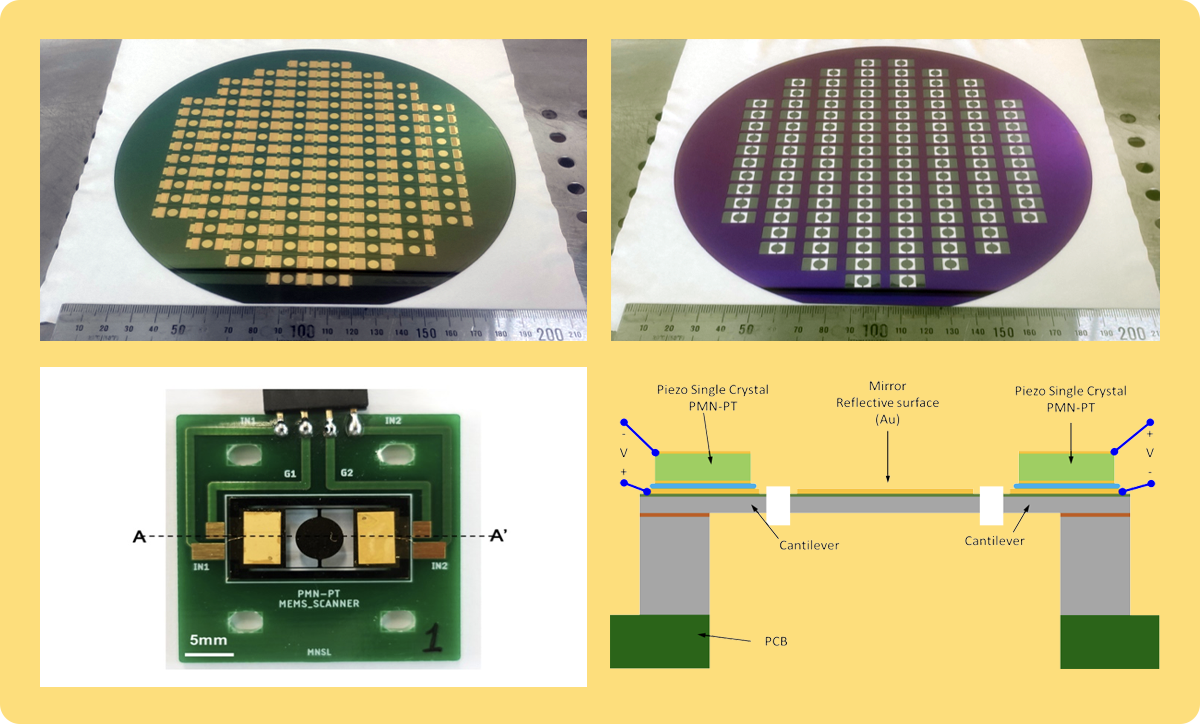•No.1

•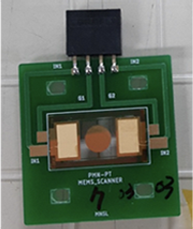No.2

•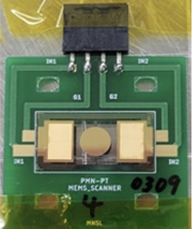No.3

•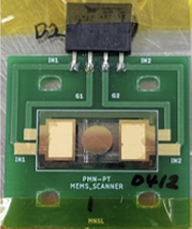No.4

•No.5

•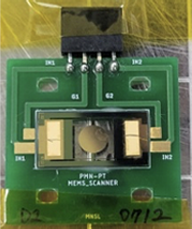No.6

Resonance Frequency [kHz] 30° Drive Voltage [Vpp]
3.5 5.5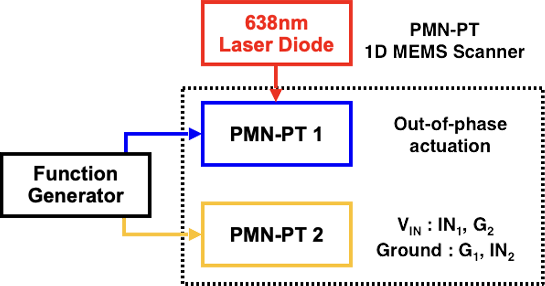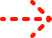Optical scan angle of 30°

### Single Crystal Properties

PMN-PT

Parameter Units  poled  poled
Low-PT High-PT Low-PT High-PT
${ϵ}_{33}T/{ϵ}_{0}$ - 4842 7000 3760 5770
${d}_{ij}$ ${\mathrm{x10}}^{-12}C/N$ ${d}_{33}=1282$ ${d}_{33}=1620$ ${d}_{33}=-1140$ ${d}_{33}=-1820$
${S}_{ij}^{E}$ ${\mathrm{x10}}^{-12}{m}^{2}/N$ ${S}_{33}^{E}=47$ ${S}_{33}^{E}=56$ ${S}_{33}^{E}=53$ ${S}_{33}^{E}=78$
Trt ${}^{0}C$ 95 85 95 85
Ec KV/cm 2 2.5 2 2.5
Density $kg/{m}^{3}$ 8080

PIN-PMN-PT

Parameter Units  poled  poled
Low-PT High-PT Low-PT High-PT
${ϵ}_{33}T/{ϵ}_{0}$ - 4457 5666 3449 4656
${d}_{ij}$ ${\mathrm{x10}}^{-12}C/N$ ${d}_{33}=1226$ ${d}_{33}=1840$ ${d}_{33}=-1323$ ${d}_{33}=-1870$
${S}_{ij}^{E}$ ${\mathrm{x10}}^{-12}{m}^{2}/N$ ${S}_{33}^{E}=49$ ${S}_{33}^{E}=79$ ${S}_{33}^{E}=72$ ${S}_{33}^{E}=99$
Trt ${}^{0}C$ 120 90 120 90
Ec KV/cm 4 6 4 6
Density $kg/{m}^{3}$ 8154